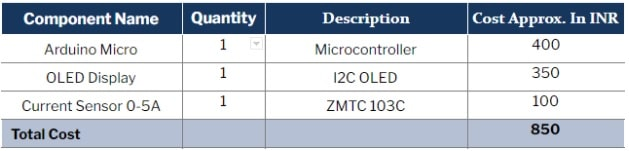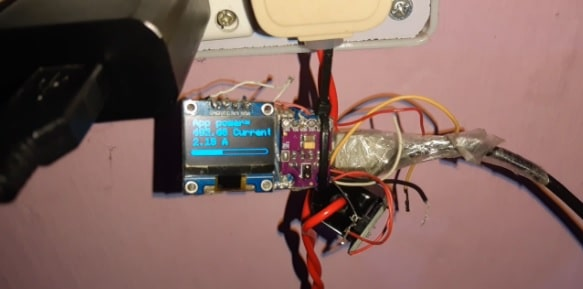AC Current Monitor Using Arduino

Ashwini Kumar Sinha

1389

Obtaining AC current readings from an AC wire helps us to know the amount of power that electrical appliances consume, promoting effective maintenance of the required load and supply.

So today you will make a current meter that performs current measurement and gives real-time data regarding the current consumed by various electrical appliances. The device also gives real- time power consumption data.

Bill Of MaterialCoding

First install the u8g2 library for OLED and sensors using the library manager in Arduino IDE. While the u8g2lib enables data display on the OLED, the emonLib facilitates in obtaining the current reading from the sensor. So include these libraries in the setup function of the code.

Begin by defining the pin number and calibration factor for the current sensor. Then create a loop function for reading the analogue value from the current sensor. After doing this, convert the obtained value into IRMS, that is, Ipeak divided by the square root of two.

Next, calculate the apparent power. It is given by:

Apparent power = Reactive power + True power

Or

P = ErmsIrms

where P is apparent power

Here voltage is 230 V and IRMS is the current reading from sensor

Therefore, Apparent Power (P) = IRMS x 230V

In the code, multiply the IRMS value (that is extracted by the sensor) with 230V.

After calculating the apparent voltage and apparent power, update the OLED display so that the detected value is shown on the screen.

Connection

Join the components as shown in the circuit diagram. Also, connect the electrical wire to sensor.